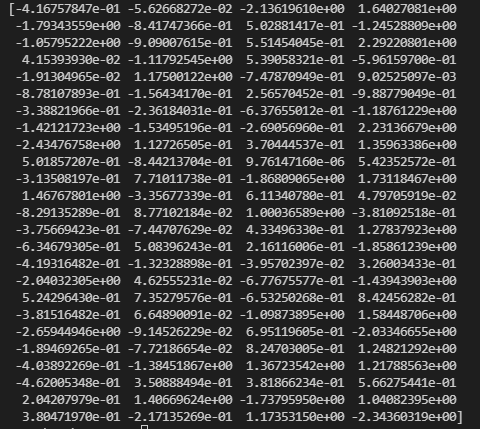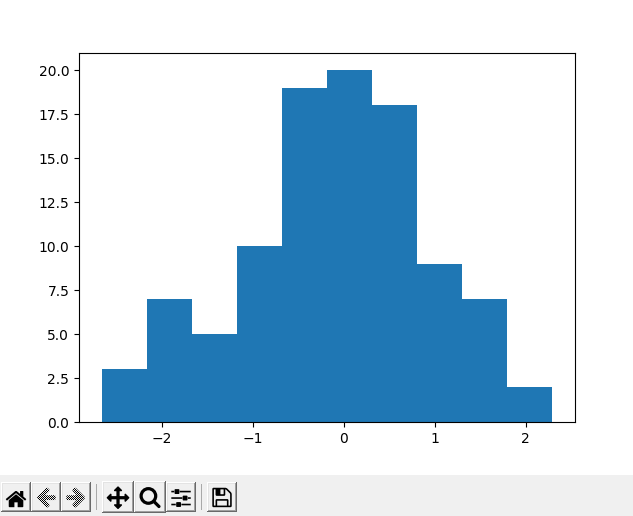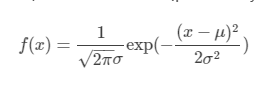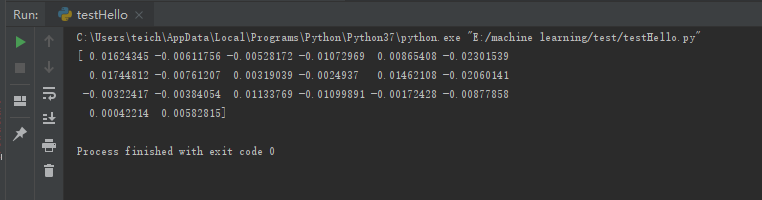• 1 np.random.normal()  normal代表的是生成正态分布，正态分布需要知道它的均值和方程。ndarray需要知道数组的大小。使用一共有3个参数。numpy.random.normal(loc=0.0, scale=1.0,size=shape) 参数loc(flo
• 主要介绍了np.random.seed() 的使用详解，文中通过示例代码介绍的非常详细，对大家的学习或者工作具有一定的参考学习价值，需要的朋友们下面随着小编来一起学习学习吧
• np.random.normal()的意思是一个正态分布 normal------>正态 例子： noise = np.random.normal(loc=0,scale=0.02,size=shape) 意义如下： 参数loc(float)：正态分布的均值，对应着这个分布的中心。loc=0说明这一...
 import numpy as np

np.random.normal()的意思是一个正态分布 normal------>正态
例子：
noise = np.random.normal(loc=0,scale=0.02,size=shape)

意义如下：
参数loc(float)：正态分布的均值，对应着这个分布的中心。loc=0说明这一个以Y轴为对称轴的正态分布， 参数scale(float)：正态分布的标准差，对应分布的宽度，scale越大，正态分布的曲线越矮胖，scale越小，曲线越高瘦。 参数size(int 或者整数元组)：输出的值赋在shape里，默认为None。
展开全文python numpy
• 一、np.random.normal()函数用法 numpy.random.normal(loc=0,scale=1e-2,size=shape) 其参数意义为： 参数loc(float)：正态分布的均值，对应着这个分布的中心。loc=0说明这一个以Y轴为对称轴的正态分布， 参数scale...
一、np.random.normal()函数用法
numpy.random.normal(loc=0,scale=1e-2,size=shape)
其参数意义为： 参数loc(float)：正态分布的均值，对应着这个分布的中心。loc=0说明这一个以Y轴为对称轴的正态分布， 参数scale(float)：正态分布的标准差，对应分布的宽度，scale越大，正态分布的曲线越矮胖，scale越小，曲线越高瘦。 参数size(int 或者整数元组)：输出的值赋在shape里，默认为None。
标准正态分布，对应于np.random.normal(loc=0, scale=1, size)
二、tf.random_normal()函数用法
tf.random_normal(shape, mean=0.0, stddev=1.0, dtype=tf.float32, seed=None, name=None)
其参数意义为： shape: 输出张量的形状，必选 mean: 正态分布的均值，默认为0 stddev: 正态分布的标准差，默认为1.0 dtype: 输出的类型，默认为tf.float32 seed: 随机数种子，是一个整数，当设置之后，每次生成的随机数都一样 name: 操作的名称
标准正态分布，对应于tf.random_normal([1,1])
参考： https://blog.csdn.net/dcrmg/article/details/79028043/ https://blog.csdn.net/qq_37701443/article/details/82797944
展开全文numpy
• data = np.random.normal(size=100) print(data) 打印： 直方图： plt.hist(data) 例： """normal：正态分布平均值9000标准差3000 300个数值""" data=np.random.normal(9000,3000,si...
默认：
numpy.random.normal（ loc=0.0 , scale=1.0 , size=None）

"""平均值0，标准差1  100个值"""
data = np.random.normal(size=100)
print(data)
打印：直方图：
plt.hist(data)例：
"""normal：正态分布 平均值9000  标准差3000 300个数值"""

data = np.random.normal(9000, 3000, size=300)

展开全文python numpy
• ## np.random.normal()

千次阅读 2019-07-14 19:30:49
先看伟大的高斯分布（Gaussian Distribution）的概率密度函数...numpy.random.normal(loc=0.0, scale=1.0, size=None) 参数的意义为： loc：float 此概率分布的均值（对应着整个分布的中心centre） scale：floa...
先看伟大的高斯分布（Gaussian Distribution）的概率密度函数（probability density function）：对应于numpy中：
numpy.random.normal(loc=0.0, scale=1.0, size=None)
参数的意义为：
loc：float
此概率分布的均值（对应着整个分布的中心centre）
scale：float
此概率分布的标准差（对应于分布的宽度，scale越大越矮胖，scale越小，越瘦高）
size：int or tuple of ints
输出的shape，默认为None，只输出一个值

我们更经常会用到的np.random.randn(size)所谓标准正态分布（μ=0,σ=1μ=0,σ=1），对应于np.random.normal(loc=0, scale=1, size)。
例子
import numpy as np
rgen = np.random.RandomState(1)
w = rgen.normal(0, 0.01, 20)
print(w)展开全文• ## np.random.normal()函数

千次阅读 2020-09-27 23:33:43
今天啃动手学习深度学习的时候，突然忘记了 np.random.normal()函数是啥用的了，记个随笔   函数原型： np.random.normal(loc=0.0, scale=1.0, size=None) 各参数意义如下： 参数 loc(float)：正态分布的...
• numpy.random.normal(loc=0.0,scale=1.0,size=None) 参数的意义为： 　loc:float 　概率分布的均值，对应着整个分布的中心center 　scale:float 　概率分布的标准差，对应于分布的宽度，scale越大越矮胖，...计算机视觉 深度学习 机器学习
• np.random.normal(loc=0.0, scale=1.0, size=None) 参数解释： loc(float)：此概率分布的均值（对应着整个分布的中心centre scale(float)：此概率分布的标准差（对应于分布的宽度，scale越大，图形越矮胖；scale越...python numpy 机器学习
• ## np.random.Normal()

千次阅读 2018-10-27 10:50:49
np.random.normal()正态分布 在numpy计算中，一般会有： np.random.normal(size,loc,scale): loc：float 此概率分布的均值（对应着整个分布的中心centre） scale：float 此概率分布的标准差（对应于分布的宽度...
• python中的np.random.normal（） np.random.normal(size,loc,scale): 给出均值为loc，标准差为scale的高斯随机数（场）. 先看伟大的高斯分布（Gaussian Distribution）的概率密度函数（probability density function...numpy
• 这是个随机产生正态分布的函数。（normal 表正态） 先看一下官方解释： 有三个参数， loc:正态分布的均值，对应着这个分布的中心....scale：正态分布的标准差，对应分布的宽度...a=np.random.normal(0, 1, (2, 4)) print(apython
• ## np.random.normal()讲解

千次阅读 2020-04-05 19:46:04
这是的np是numpy包的缩写，np.random.normal()的意思是一个正态分布，numpy.random.normal(loc=0,scale=1e-2,size=shape) ，意义如下： 参数loc(float)：正态分布的均值，对应着这个分布的中心。loc=0说明这一个以Y...
• 参考文献：Python学习之np.random.normal()函数. A np.random.normal()的API numpy.random.normal(loc=0,scale=1e-2,size=shape) 参数loc(float)：正态分布的均值。 参数scale(float)：正态分布的标准差。 参数size...python numpy
• ## Python学习之np.random.normal()函数

万次阅读 多人点赞 2018-06-21 16:15:01
这是的np是numpy包的缩写，np.random.normal()的意思是一个正态分布，normal这里是正态的意思。 我在看孪生网络的时候看到这样的一个例子：numpy.random.normal(loc=0,scale=1e-2,size=shape) ，意义如下： 参数...numpy
• np.random.normal() https://blog.csdn.net/lanchunhui/article/details/50163669 numpy.random.normal(loc, scale, size) loc：float 此概率分布的均值（对应着整个分布的中心centre） scale：float 此概率分布...
• np.cumsum() 累计求和，示例如下：&gt;&gt;&gt; a = np.array([[1,2,3], [4,5,6]])&gt;&gt;&gt; aarray([[1, 2, 3], [4, 5, 6]])&gt;&gt;&gt; np.cumsum(a)array(...
• python中numpy.random.normal(loc=0,scale=1e-2,size=shape) ，意义如下： 参数loc(float)：正态分布的均值，对应着这个分布的中心。loc=0说明这一个以Y轴为对称轴的正态分布， 参数scale(float)：正态分布的标准...python 机器学习 numpy 人工智能 深度学习
• np.random.normal()正态分布 在numpy计算中，一般会有： np.random.normal(size,loc,scale): loc：float 此概率分布的均值（对应着整个分布的中心centre）scale：float 此概率分布的标准差（对应于分布的...
• 目录1、numpy.random.uniform()2、np.random.randn()3、np.random.binomial() 1、numpy.random.uniform() numpy.random.uniform(low,high,size) 从一个均匀分布[low,high)中随机采样，注意定义域是左闭右开，即包含...
• numpy.random.normal(loc=0.0, scale=1.0, size=None) 参数的意义为： loc：float  此概率分布的均值（对应着整个分布的中心centre） scale：float  此概率分布的标准差（对应于分布的宽度，scale越大越矮胖，......